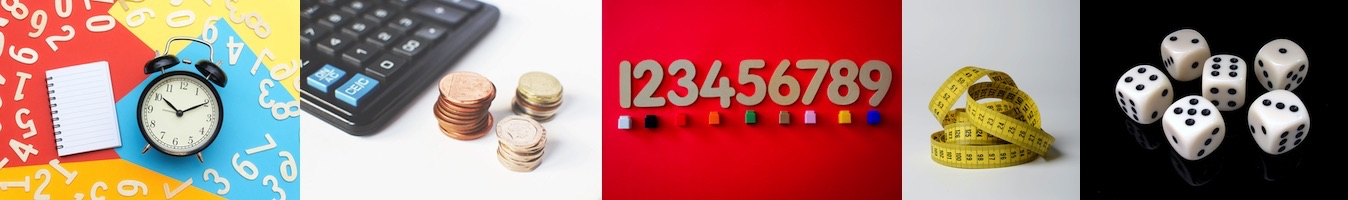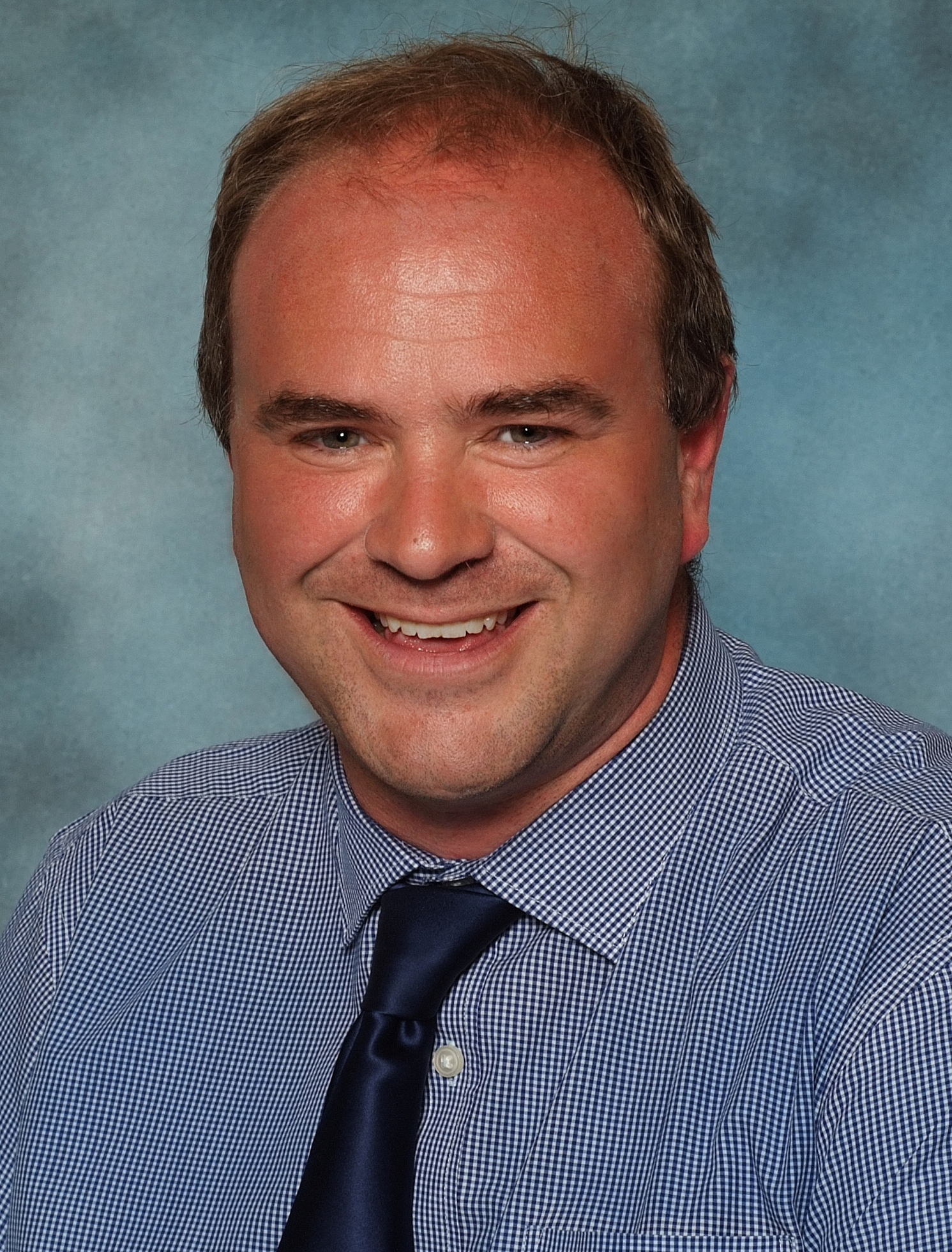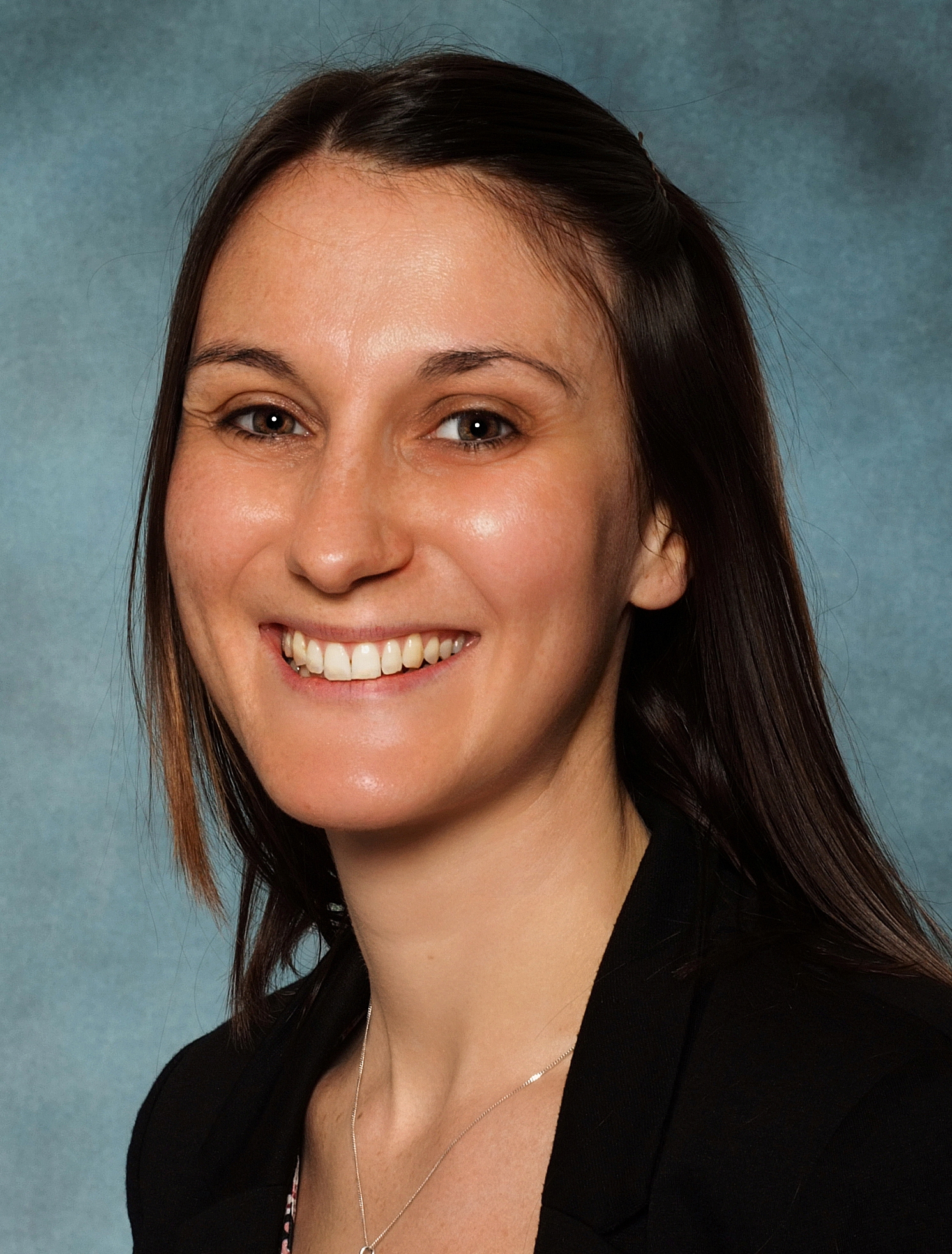Select Page# Maths#### Our Lower School MathsSubject Leader is Mr Dodd#### Maths in Lower School

In Maths I will learn to read, write, estimate and count with whole numbers within and above one hundred.  I will be able to work with both numerals and words and share my knowledge and understanding using objects, pictorial representations, number lines, and precise mathematical vocabulary.   My place value knowledge will grow and I will be able to partition two-digit numbers, allowing me to successfully solve place value problems.

Using concrete objects and pictorial representations, I will learn to solve addition and subtraction problems and then apply my increasing knowledge to mental and written methods.  I will become fluent in number bonds to 10 and 20 and use these to derive related facts up to 100.  I will be taught to solve problems involving multiplication and division.  I will learn to count in twos, fives and tens and identify the connections between them.  I will begin to show precision and fluency in the related division facts.  By the end of Year 2, I will be able to recognise, find and name some simple unit and non-unit fractions.

Using practical resources, I will develop my ability to recognise, describe, draw, compare and sort different 2D and 3D shapes and use the related mathematical vocabulary.   I will be able to I will use standard units of measurement with increasing accuracy, using my knowledge of the number system. I will be able to use measures to describe and compare different quantities such as length, mass, capacity/volume, time and money.

#### Maths in Upper School

In Maths I will develop a secure knowledge of place value and become fluent with larger whole numbers, number facts, and decimal numbers.   This will allow me to become efficient and successful at using both mental and written methods of calculation for all four operations.  I will learn to effectively solve mathematical problems with increasing complexity and use my knowledge to make reasonable explanations and judgements.  I will be introduced to algebra as a means for solving a variety of problems.

I will learn my multiplication tables up to and including the 12 multiplication table and show precision and fluency in the related division facts.   I will learn to make connections between multiplication and division with fractions, decimals, percentages and ratio.

I will be able to classify, analyse and confidently describe shapes and their properties and the relationships between them using the appropriate vocabulary.  I will be able to accurately use a range of measuring equipment and make connections between measure and the number facts that I have learned.

I will be able to use the skills that I learn in Maths, in my Science, Technology and Computing work.

#### Curriculum Map

Below is a link to our Curriculum Map for Maths.

The curriculum map ensures that every child receives their full curriculum entitlement as they progress through the school.

We plan and deliver our curriculum on a one year cycle.

Curriculum Map (Maths)